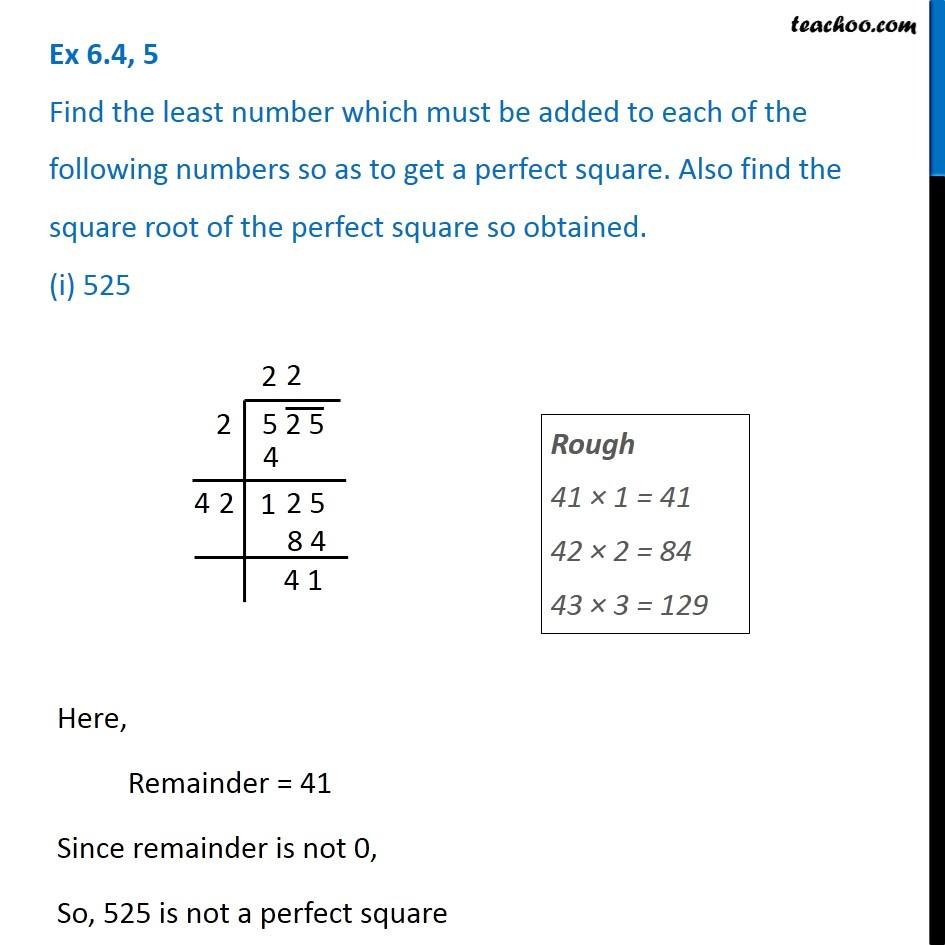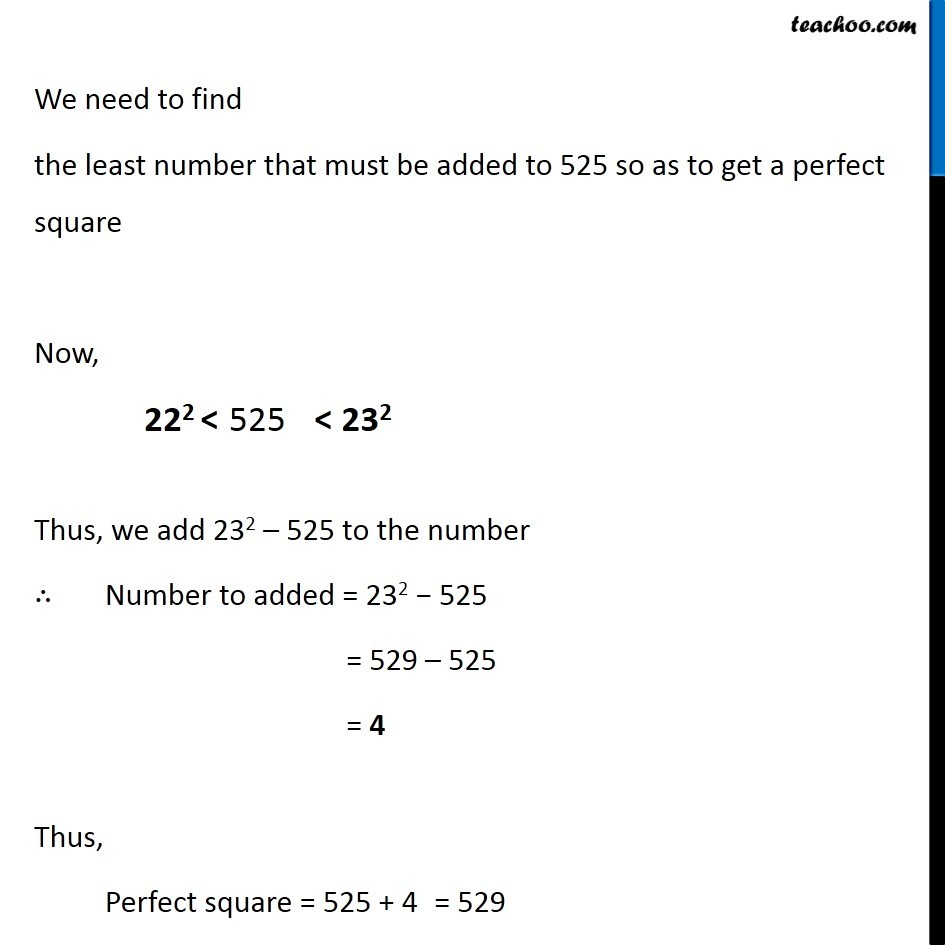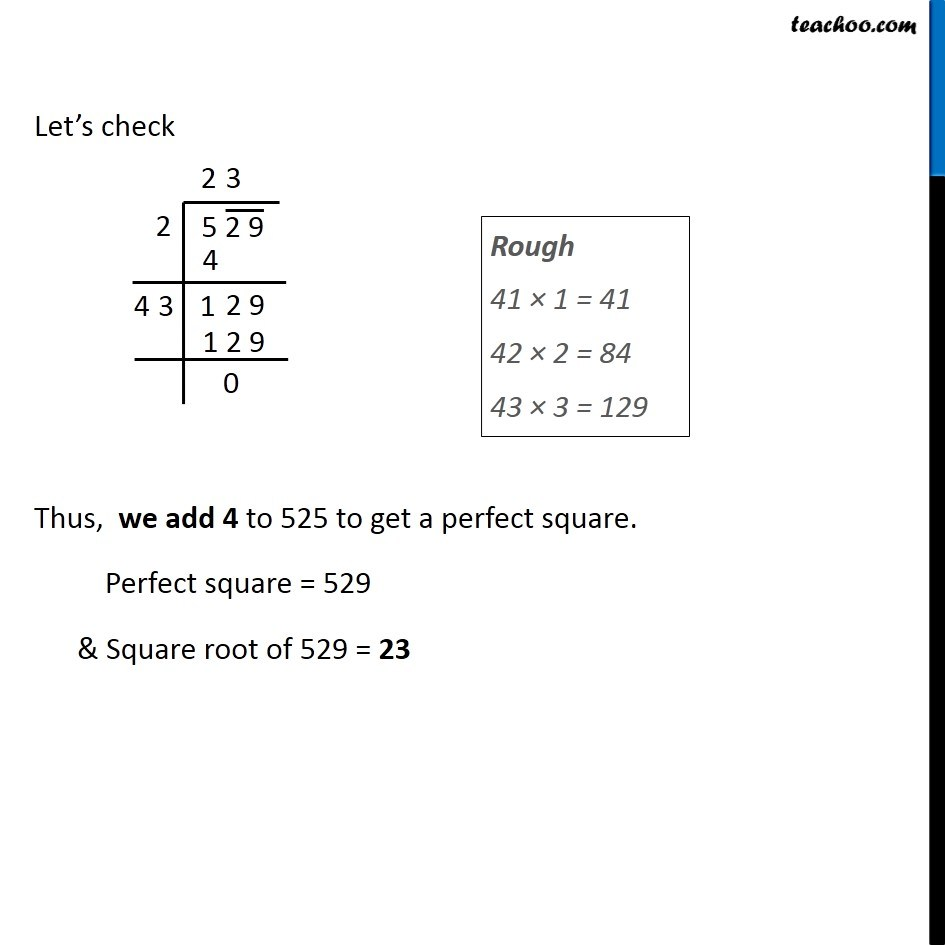Ex 5.4

Chapter 5 Class 8 Squares and Square Roots
Serial order wiseLearn in your speed, with individual attention - Teachoo Maths 1-on-1 Class

### Transcript

Ex 5.4, 5 Find the least number which must be added to each of the following numbers so as to get a perfect square. Also find the square root of the perfect square so obtained. (i) 525Rough 41 × 1 = 41 42 × 2 = 84 43 × 3 = 129 Here, Remainder = 41 Since remainder is not 0, So, 525 is not a perfect square We need to find the least number that must be added to 525 so as to get a perfect square Now, Thus, we add 232 – 525 to the number ∴ Number to added = 232 − 525 = 529 – 525 = 4 Thus, Perfect square = 525 + 4 Let’s check Thus, we add 4 to 525 to get a perfect square. Perfect square = 529 & Square root of 529 = 23 Rough 41 × 1 = 41 42 × 2 = 84 43 × 3 = 129